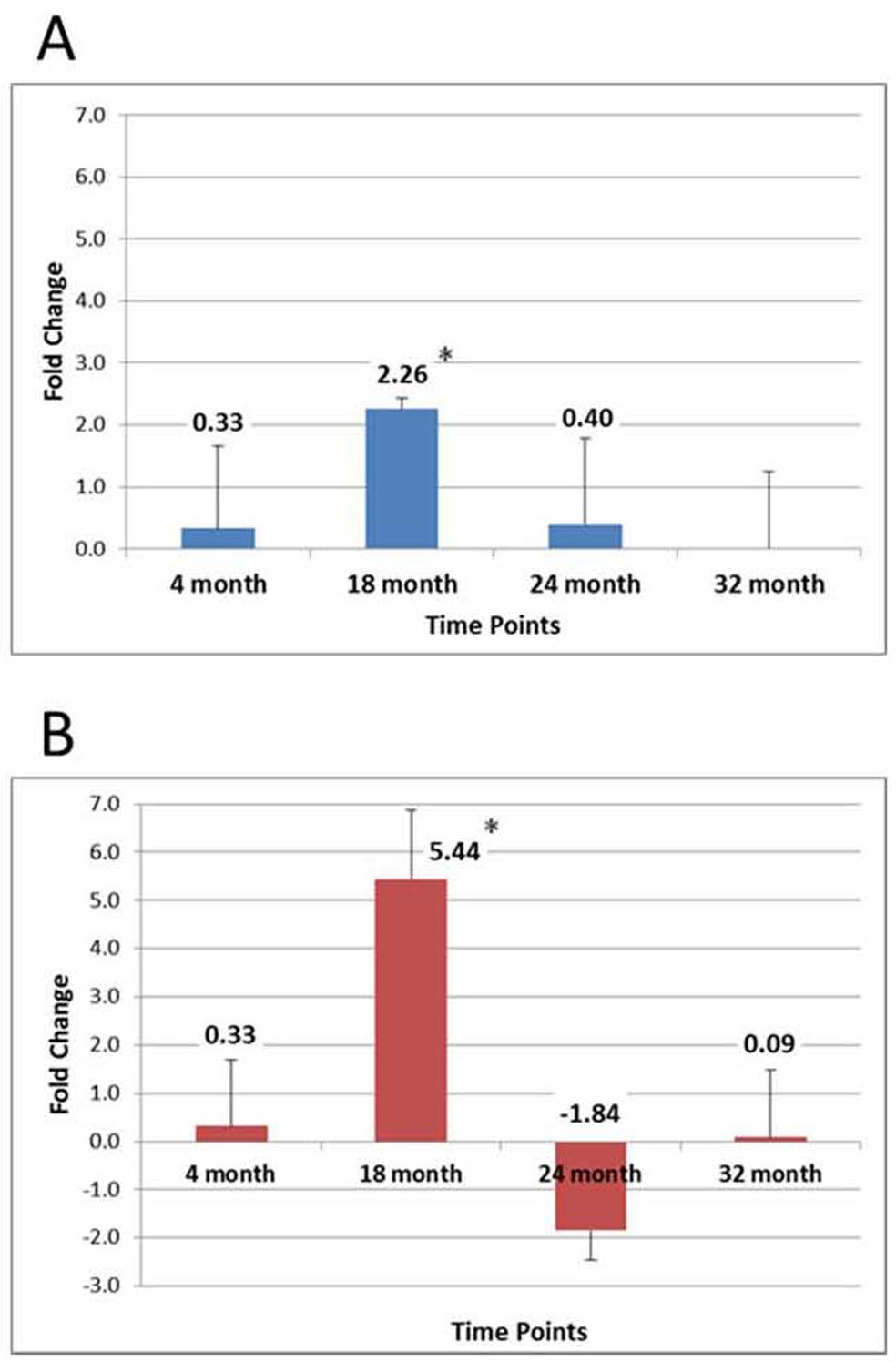Figure 4 of Smit-McBride, Mol Vis 2014; 20:1569-1578.

Figure 4. Real-time PCR (RT-PCR) results for the expression of miR-34a target mRNA Sirt1 in the aging posterior mouse eye. RT–PCR of the retina (A) and RPE/choroid (B) isolated total RNA samples at 4, 18, 24, and 32 months of age. There were three samples (n=3) for each of the four time points, for a total of twelve animals (n=12). Total RNA samples from each animal tissue at each time point were run in triplicate, resulting in each time point representing an average of nine data values. Data were normalized to a geometric mean of the three control genes (Gapdh, B2M, and Hprt1) and calibrated to the 4-month-old sample. The y-axis represents the fold change compared to the 4 month old. * denotes statistically significant differences (p<0.01). Error bars represent standard deviation (SD) values.